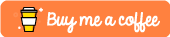# #Python – Working with dates 📅, formats, and subtract for time difference. Timedelta type rocks!Hi !

I’m switching from C#9 to Python to write this down, so I avoid to search and write this from scratch again and again. My scenario includes very simple operations with dates,

• Tag a start date time
• Do some process, I’ll fake it with random sleep
• Tag a end date time
• Calculate the different between them
• Process and show the difference with a specific format

For this I’ll use the datetime type, and a cool feature using datetime is that we can directly substract 2 dates. In example:

```start = datetime.datetime.utcnow()
time.sleep(10)
end = datetime.datetime.utcnow()

delta = end - start
```

In this code 👆, the delta variable is a timedelta type. This is not a standard datetime, in C# we know this as TimeSpan. We can access the internal values, and get some details around hours, minutes, seconds and millisecons:

```def get_timedelta_values(delta):
# Get the hours, minutes, seconds and milliseconds
millis           = round(delta.microseconds/1000, 0)
minutes, seconds = divmod(delta.seconds, 60)
hours, minutes   = divmod(minutes, 60)
return hours, minutes, seconds, millis

def get_timedelta_min_and_sec(delta):
hours, minutes, seconds, millis = get_timedelta_values(delta)
return str(f'{str(minutes).zfill(2)}:{str(seconds).zfill(2)}')
```

The 2nd function, get the delta values and creates a standard output with “mm:ss” format. So, not tricky and easy to move forward.

Another cool feature of the timedelta is that support adding. So as a final example, I’ll generate some random deltas in a loop, and add them in a totalTime var.

```import time
import datetime
from random import randint

def get_timedelta_values(delta):
# Get the hours, minutes, seconds and milliseconds
millis           = round(delta.microseconds/1000, 0)
minutes, seconds = divmod(delta.seconds, 60)
hours, minutes   = divmod(minutes, 60)
return hours, minutes, seconds, millis

def get_timedelta_min_and_sec(delta):
hours, minutes, seconds, millis = get_timedelta_values(delta)
return str(f'{str(minutes).zfill(2)}:{str(seconds).zfill(2)}')

totalTime = None
i = 0
while i < 25:
i = i + 1
rndSleep = randint(1, 10)
print(f'current iteration: {i} - rnd sleep: {rndSleep}')

start = datetime.datetime.utcnow()
time.sleep(rndSleep)
end = datetime.datetime.utcnow()

delta = end - start

if (totalTime is None):
totalTime = delta
else:
totalTime += delta

print(f'  >> delta     : {get_timedelta_min_and_sec(delta)}')
print(f'  >> total time: {get_timedelta_min_and_sec(totalTime)}')
```

The output as expected shows the delta with specific format in each iteration and also the accumulator for the total time

So, here it is, a simple example that will save me some time in the future for sure !

Happy coding!

Greetings

El Bruno